# Recent Activity

## Universal Steiner triple systems ★★

Author(s): Grannell; Griggs; Knor; Skoviera

Problem   Which Steiner triple systems are universal?

Keywords: cubic graph; Steiner triple system

## Monotone 4-term Arithmetic Progressions ★★

Author(s): Davis; Entringer; Graham; Simmons

Question   Is it true that every permutation of positive integers must contain monotone 4-term arithmetic progressions?

## The Bermond-Thomassen Conjecture ★★

Author(s): Bermond; Thomassen

Conjecture   For every positive integer, every digraph with minimum out-degree at least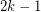containsdisjoint cycles.

Keywords: cycles

## Continous analogue of Hirsch conjecture ★★

Author(s): Deza; Terlaky; Zinchenko

Conjecture   The order of the largest total curvature of the primal central path over all polytopes defined byinequalities in dimensionis.

Keywords: curvature; polytope

## Average diameter of a bounded cell of a simple arrangement ★★

Author(s): Deza; Terlaky; Zinchenko

Conjecture   The average diameter of a bounded cell of a simple arrangement defined byhyperplanes in dimensionis not greater than.

Keywords: arrangement; diameter; polytope

## Drawing disconnected graphs on surfaces ★★

Author(s): DeVos; Mohar; Samal

Conjecture   Letbe the disjoint union of the graphs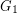andand let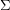be a surface. Is it true that every optimal drawing ofonhas the property thatandare disjoint?

Keywords: crossing number; surface

## Matchings extend to Hamiltonian cycles in hypercubes ★★

Author(s): Ruskey; Savage

Question   Does every matching of hypercube extend to a Hamiltonian cycle?

Keywords: Hamiltonian cycle; hypercube; matching

## Linear Hypergraphs with Dimension 3 ★★

Author(s): de Fraysseix; Ossona de Mendez; Rosenstiehl

Conjecture   Any linear hypergraph with incidence poset of dimension at most 3 is the intersection hypergraph of a family of triangles and segments in the plane.

Keywords: Hypergraphs

## Reed's omega, delta, and chi conjecture ★★★

Author(s): Reed

For a graph, we define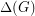to be the maximum degree,to be the size of the largest clique subgraph, andto be the chromatic number of.

Conjecture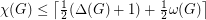for every graph.

Keywords: coloring

## Pebbling a cartesian product ★★★

Author(s): Graham

We let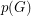denote the pebbling number of a graph.

Conjecture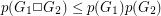.

Keywords: pebbling; zero sum

## Rendezvous on a line ★★★

Author(s): Alpern

Problem   Two players start at a distance of 2 on an (undirected) line (so, neither player knows the direction of the other) and both move at a maximum speed of 1. What is the infimum expected meeting time(first time when the players occupy the same point) which can be achieved assuming the two players must adopt the same strategy?

Keywords: game theory; optimization; rendezvous

## Linial-Berge path partition duality ★★★

Author(s): Berge; Linial

Conjecture   The minimum-norm of a path partition on a directed graph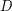is no more than the maximal size of an induced-colorable subgraph.

Keywords: coloring; directed path; partition

## What is the smallest number of disjoint spanning trees made a graph Hamiltonian ★★

Author(s): Goldengorin

We are given a complete simple undirected weighted graph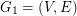and its first arbitrary shortest spanning tree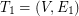. We define the next graph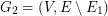and find onthe second arbitrary shortest spanning tree. We continue similarly by findingon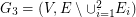, etc. Let k be the smallest number of disjoint shortest spanning trees as defined above and letbe the graph obtained as union of alldisjoint trees.

Question 1. What is the smallest number of disjoint spanning trees creates a graphcontaining a Hamiltonian path.

Question 2. What is the smallest number of disjoint spanning trees creates a graphcontaining a shortest Hamiltonian path?

Questions 3 and 4. Replace in questions 1 and 2 a shortest spanning tree by a 1-tree. What is the smallest number of disjoint 1-trees creates a Hamiltonian graph? What is the smallest number of disjoint 1-trees creates a graph containing a shortest Hamiltonian cycle?

Keywords: 1-trees; cycle; Hamitonian path; spanning trees

## Davenport's constant ★★★

Author(s):

For a finite (additive) abelian group, the Davenport constant of, denoted, is the smallest integerso that every sequence of elements ofwith length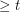has a nontrivial subsequence which sums to zero.

Conjecture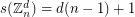Keywords: Davenport constant; subsequence sum; zero sum

## Coloring and immersion ★★★

Author(s): Abu-Khzam; Langston

Conjecture   For every positive integer, every (loopless) graphwithimmerses.

Keywords: coloring; complete graph; immersion

## Rainbow AP(4) in an almost equinumerous coloring ★★

Author(s): Conlon

Problem   Do 4-colorings of, fora large prime, always contain a rainbow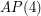if each of the color classes is of size of eitheror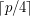?

Keywords: arithmetic progression; rainbow

## The intersection of two perfect matchings ★★

Author(s): Macajova; Skoviera

Conjecture   Every bridgeless cubic graph has two perfect matchings,so thatdoes not contain an odd edge-cut.

Keywords: cubic; nowhere-zero flow; perfect matching

## Counterexamples to the Baillie-PSW primality test ★★

Author(s):

Problem  (1)   Find a counterexample to Baillie-PSW primality test or prove that there is no one.
Problem  (2)   Find a composite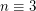or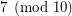which divides both(see Fermat pseudoprime) and the Fibonacci number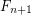(see Lucas pseudoprime), or prove that there is no such.

Keywords:

## A sextic counterexample to Euler's sum of powers conjecture ★★

Author(s): Euler

Problem   Find six positive integers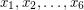such thator prove that such integers do not exist.

Keywords:

## Geodesic cycles and Tutte's Theorem ★★

Author(s): Georgakopoulos; Sprüssel

Problem   Ifis a-connected finite graph, is there an assignment of lengths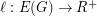to the edges of, such that every-geodesic cycle is peripheral?

Keywords: cycle space; geodesic cycles; peripheral cycles